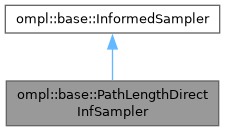ompl::base::PathLengthDirectInfSampler Class Reference

An informed sampler for problems seeking to minimize path length. More...

#include <ompl/base/samplers/informed/PathLengthDirectInfSampler.h>

Inheritance diagram for ompl::base::PathLengthDirectInfSampler:[legend]

## Public Member Functions

PathLengthDirectInfSampler (const ProblemDefinitionPtr &probDefn, unsigned int maxNumberCalls)
Construct a sampler that only generates states with a heuristic solution estimate that is less than the cost of the current solution using a direct ellipsoidal method. More...

bool sampleUniform (State *statePtr, const Cost &maxCost) override
Sample uniformly in the subset of the state space whose heuristic solution estimates are less than the provided cost, i.e. in the interval [0, maxCost). Returns false if such a state was not found in the specified number of iterations.

bool sampleUniform (State *statePtr, const Cost &minCost, const Cost &maxCost) override
Sample uniformly in the subset of the state space whose heuristic solution estimates are between the provided costs, [minCost, maxCost). Returns false if such a state was not found in the specified number of iterations.

bool hasInformedMeasure () const override
Whether the sampler can provide a measure of the informed subset.

double getInformedMeasure (const Cost &currentCost) const override
The measure of the subset of the state space defined by the current solution cost that is being searched. Does not consider problem boundaries but returns the measure of the entire space if no solution has been found. In the case of multiple goals, this measure assume each individual subset is independent, therefore the resulting measure will be an overestimate if any of the subsets overlap.

Cost heuristicSolnCost (const State *statePtr) const override
A helper function to calculate the heuristic estimate of the solution cost for the informed subset of a given state. More...Public Member Functions inherited from ompl::base::InformedSampler
InformedSampler (const InformedSampler &)=delete

InformedSampleroperator= (const InformedSampler &)=delete

InformedSampler (const ProblemDefinitionPtr &probDefn, unsigned int maxNumberCalls)
Construct a sampler that only generates states with a heuristic solution estimate that is less than the cost of the current solution. Requires a function pointer to a method to query the cost of the current solution. If iteration is required, only maxNumberCalls are attempted, to assure that the function returns.

virtual double getInformedMeasure (const Cost &minCost, const Cost &maxCost) const
The measure of the subset of the state space defined by the current solution cost that is being searched. Does not consider problem boundaries but returns the measure of the entire space if no solution has been found or if a closed form expression for the measure does not exist. By default calls the 1-argument overloaded version with the min and max costs and subtracts the differences; however, there may be more efficient ways to do this for some cost functions.

ProblemDefinitionPtr getProblemDefn () const

unsigned int getMaxNumberOfIters () constProtected Attributes inherited from ompl::base::InformedSampler
ProblemDefinitionPtr probDefn_
A copy of the problem definition.

OptimizationObjectivePtr opt_
A copy of the optimization objective.

StateSpacePtr space_
A copy of the state space.

unsigned int numIters_
The number of iterations I'm allowed to attempt.

## Detailed Description

An informed sampler for problems seeking to minimize path length.

PathLengthDirectInfSampler is a method to generate uniform samples in the subset of a problem that could provide a shorter path from start to goal. This subset is a prolate hyperspheroid (PHS), a special type of an hyperellipsoid) and can be sampled directly.

Informed sampling is a method to focus search which continuing to consider all homotopy classes that can provide a better solution. Directly sampling the informed subset guarantees a non-zero probability of improving a solution regardless of the size of the planning domain, the number of state dimensions, and how close the current solution is to the theoretical minimum.

Currently only implemented for problems in R^n (i.e., RealVectorStateSpace), SE(2) (i.e., SE2StateSpace), and SE(3) (i.e., SE3StateSpace). Until an initial solution is found, this sampler simply passes-through to a uniform distribution over the entire state space.

Associated publication:

J. D. Gammell, T. D. Barfoot, S. S. Srinivasa, "Informed sampling for asymptotically optimal path planning." IEEE Transactions on Robotics (T-RO), 34(4): 966-984, Aug. 2018. DOI: TRO.2018.2830331. arXiv: 1706.06454 [cs.RO]. Illustration video. Short description video.

Definition at line 144 of file PathLengthDirectInfSampler.h.

## ◆ PathLengthDirectInfSampler()

 ompl::base::PathLengthDirectInfSampler::PathLengthDirectInfSampler ( const ProblemDefinitionPtr & probDefn, unsigned int maxNumberCalls )

Construct a sampler that only generates states with a heuristic solution estimate that is less than the cost of the current solution using a direct ellipsoidal method.

Note: We don't check that there is a cost-to-go heuristic set in the optimization objective, as this direct sampling is only for Euclidean distance.

Definition at line 122 of file PathLengthDirectInfSampler.cpp.

## ◆ heuristicSolnCost()

 Cost ompl::base::PathLengthDirectInfSampler::heuristicSolnCost ( const State * statePtr ) const
overridevirtual

A helper function to calculate the heuristic estimate of the solution cost for the informed subset of a given state.

Todo:
Use a heuristic function for the full solution cost defined in OptimizationObjective or some new Heuristic class once said function is defined.

Reimplemented from ompl::base::InformedSampler.

Definition at line 409 of file PathLengthDirectInfSampler.cpp.

The documentation for this class was generated from the following files: